# MCQ Questions for Class 9 Maths Chapter 1 Number System with Answers

## MCQ Questions for Class 9 Maths Chapter 1 Number System with Answers

MCQs from Class 9 Maths Chapter 1 – Number System are provided here to help students prepare for their upcoming Maths exam.

MCQs from CBSE Class 9 Maths Chapter 1: Number System

1. Can we write 0 in the form of p/q?
a. Yes
b. No
c. Cannot be explained
d. None of the above

Explanation: 0 is a rational number and hence it can be written in the form of p/q.

Example: 0/4 = 0

2.) The three rational numbers between 3 and 4 are:
a. 5/2,6/2,7/2
b. 13/4,14/4,15/4
c. 12/7,13/7,14/7
d.11/4,12/4,13/4

Explanation: There are many rational numbers in between 3 and 4

To find 3 rational numbers, we need to multiply and divide both the numbers by 3+1 = 4

Hence, 3 x (4/4) = 12/4 and 4 x (4/4) = 16/4

Thus, three rational numbers between 12/4 and 16/4 are 13/4, 14/4 and 15/4.

3.) In between any two numbers there are:
a. Only one rational number
b. Many rational numbers
c. Infinite rational numbers
d. No rational number

Explanation: Take the reference from question number 2 explained above.

4.) Every rational number is:
a. Whole number
b. Natural number
c. Integer
d. Real number

5.) √9 is a __________ number.
a. Rational
b. Irrational
c. Neither rational or irrational
d. None of the above

Explanation: √9 = 3. Hence, 3 is a rational number.

6.) Which of the following is an irrational number?
a. √16
b. √(12/3)
c. √12
d. √100

Explanation: √12 cannot be simplified to a rational number.

7.) 3√6 + 4√6 is equal to:
a. 6√6
b. 7√6
c. 4√12
d. 7√12

Explanation: 3√6 + 4√6 = (3+4)√6 = 7√6

8.) √6 x √27 is equal to:
a. 9√2
b. 3√3
c. 2√2
d. 9√3

Explanation: √6 x √27 = √(6 x27) = √(2x3x3x3x3) = 3×3√2 = 9√2

9.) Which of the following is equal to x3?
a. x6-x3
b. x6.x3
c. x6/x3
d. (x6)3

Explanation: x6/x3 = x6-3 = x3

10.) Which of the following are irrational numbers?
a. √23
b. √225
c. 0.3796
d. 7.478478

Explanation: √23 = 4.79583152331…

Since the number is non-terminating non-recurring, hence, it is an irrational number.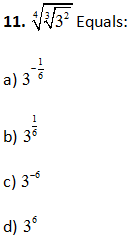12.) The product of a rational and an irrational numbers is:
a) Always an integer
b) Always a rational number
c) Always an irrational number
d) Sometimes rational and sometimes irrational

(c)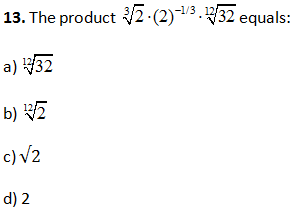(a)

14.) A rational number between √2 and √3:
a) 1.9
b) (√2. √3)/2
c) 1.5
d) 1.8

(c)

15.)Which of the following is irrational?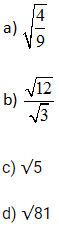(c)

16.) 4√5 + 5√5 is equal to:
a) 9√5
b) 9√10
c) 5√10
d) 7√5

(a) (ii) and (iii)

17.) Which of the following is irrational?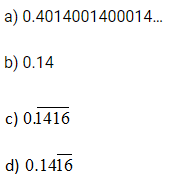(a)

18.)√12 X √15 is equal to:
a) 5√6
b) 6√5
c) 10√5
d) √25

(b)

19.) Which of the following is equal to x2?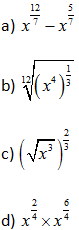(d)

20.)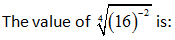a) 1/4
b) 1/2
c) 4
d) 1/16

(b)

21.) In between two rational number there is/are:
a) Exactly one rational number
b) Infinitely many rational number
c) Many irrational numbers
d) Only irrational numbers

(b)

22.) What would be the denominator after rationalizing 7/(5√3 – 5√2)?
a) 19
b) 20
c) 25
d) None of these

(d)

23.) The decimal expansion of an irrational number may be:
a) Terminating
b) Recurring
c) Either terminating or non- terminating
d) Non-terminating and non-recurring

(d)

24.)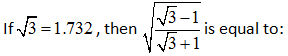a) 2.732
b) 0.2679
c) 0.732
d) 0.517

Answe

(d)

25.) Value of (256)0.16 X (256)0.09 is:
a) 4
b) 16
c) 64
d) 256.25